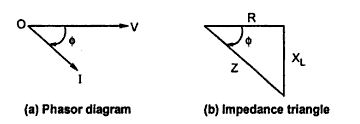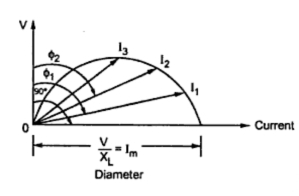### Circle Diagram for a Series R-L Circuit

Consider a series R-L circuit with a variable R as shown in the Fig. 1. It is excited by an alternating source of V volts. The frequency of the source is f Hz.Fig. 1

Let             I = Current flowing through the circuit
Z = Impedance of the circuit
Z = R + j XL        where XL = 2 fL
Now R is variable while XL is fixed.
The phasor diagram is shown in the Fig. 2(a). The current I lags voltage V by angle as the circuit is inductive. The impedance triangle is shown in the Fig. 2(b).Fig. 2

From the impedance triangle we can write,
sin Φ = XL/Z
Substituting in the expression for I,
I = (V/XL) sinΦ                                              ..............(1)
This is the equation of a circle in polar co-ordinates with a diameter equal to (V/XL).
When the resistance R =0, then Φ = 90o hence sin Φ  = 1.
...             I = Im = (V/XL)
This is the maximum value of current.
As R resistance, the phase angle decreases thus decreasing sin. Effectively current I also decrease. When R ∞ the Φ0o and current becomes zero.
The locus obtained of extremities of a current phasor plotted for various values of R is a semicircle. The semicircle is shown in the Fig. 3. The voltage axis is taken as vertical axis as a reference, with respect to which the various current phasors are plotted.Fig.  3  Circle diagram

The power factors at various conditions are cosΦ1, cosΦ2 etc. As Φ varies only from 0oto 90o, the diagram is semicircle, infact it is a half part of a circle hence it is known as circle diagram.
This theory of series R-L circuit can be easily extended to a three phase induction motor.

Related articles :